# How to use discounted cash flow for real estate valuation?

• Home
• /
• Blog
• /
• How to use discounted cash flow for real estate valuation?

# Discounted cash flow analysis in real estate

Discounted cash flow analysis (DCF) is one of the most important tools in a real estate investor's toolbox. This guide will teach you everything you need to know about DCF, from the basics to more advanced concepts.

We'll go over how to do a DCF analysis on a property, how to use the results to make investment decisions, and more. So whether you're a beginner or an experienced investor, this guide has something for you!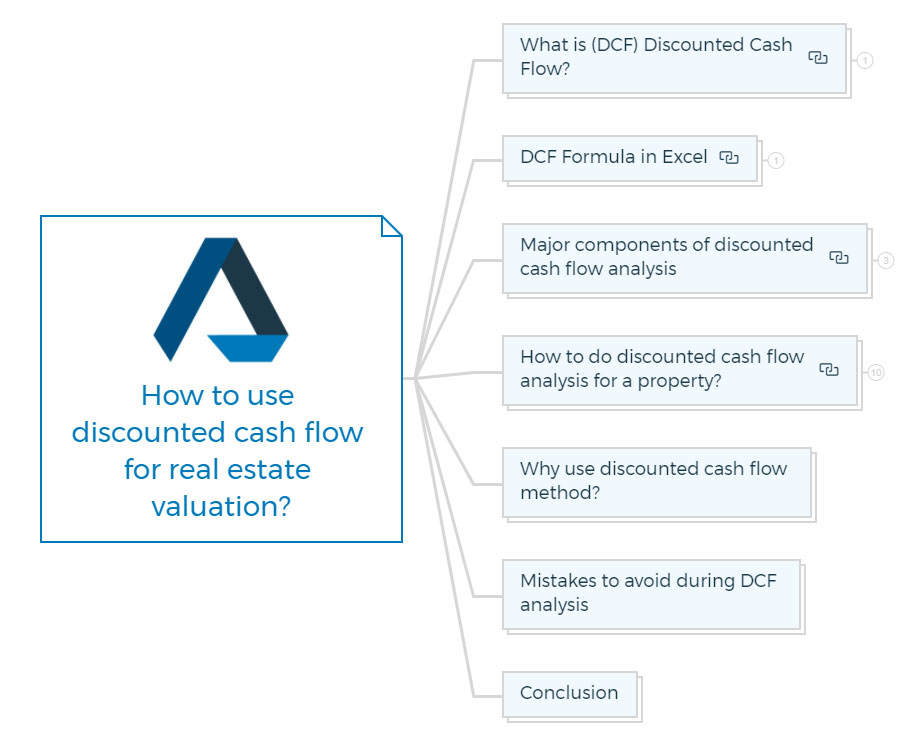#### Key Takeaways

• Discounted cash flow analysis is a powerful tool that you can use to value a wide range of investments, including real estate.
• The Discount Rate used in DCF analysis should reflect the required rate of return for a similar investment.
• Future cash flows should be estimated realistically, and all costs associated with an investment should be included.

## What is (DCF) Discounted Cash Flow?

Discounted cash flow (DCF) is a method of valuing a property or investment by Discounting future cash flows to their present value. In other words, it's a way of estimating what an investment is worth today based on its expected future cash flows.

Discounting future cash flows is important because it considers the time value of money - the idea that a dollar today is worth more than a dollar in the future.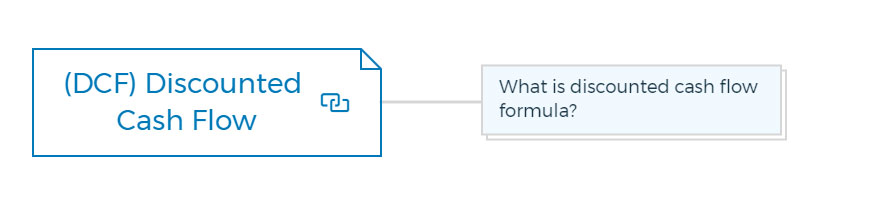### What is discounted cash flow formula?

The sum of the cash flows in each period divided by one plus the discount rate (Weighted average cost of capital) raised to the power of the period number equals the discounted cash flow (DCF) real estate formula.

The discounted cash flows formula is -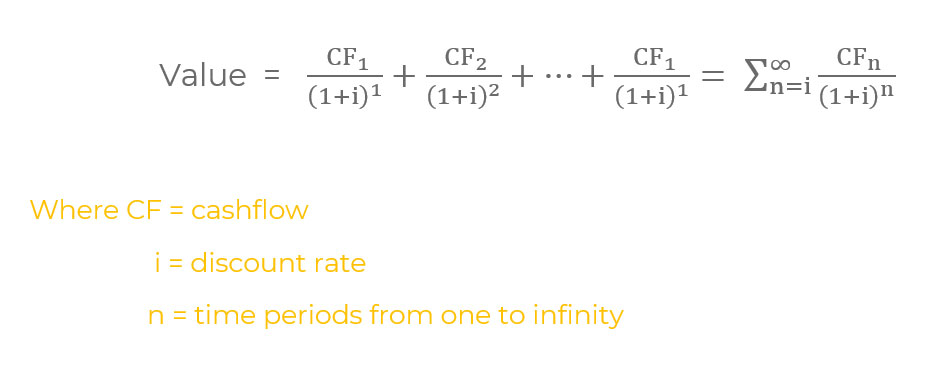WACC - WACC is the Discount Rate. The weighted average cost of capital (WACC) is a financial metric that reflects a company's overall cost of financing. WACC is the average of each type of capital cost, weighted by its proportion in the company's capital structure.

It isn't easy to manage time that never ends while remaining sensible in the real world. So, when using the DCF analysis approach to assess a company, the distant future is often integrated into one value representing the projected sale price (terminal value) at a future stage.

When the time t is substituted for infinity in the actual DCF formula, the formula is updated as below.

DCF Terminal value formula -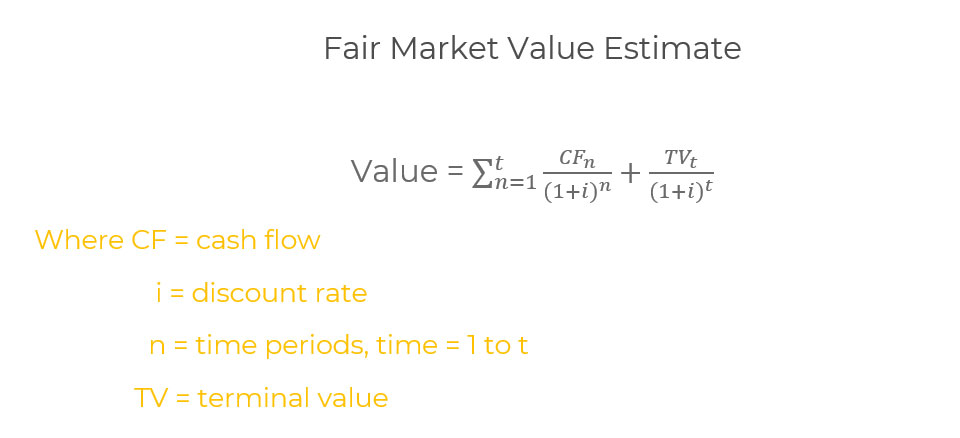Individual cash flows (CF1, CF2,..., CFt) are estimated for 5 or 10 years, and then the company's value is estimated (TVt). These estimations are then compounded to present values at the valuation date, and the present values are totalled up to get a valuation.

## DCF Formula in ExcelMicrosoft Excel provides two DCF real estate formulas for calculating discounted cash flows, which it refers to as "NPV."

The standard Net present value formula is -

=NPV(discount rate, series of cash flows)

This method assumes that all cash flows are distributed evenly overtime periods of equal length, such as years, quarters, or months. The discount rate must match the cash flows periods; for example, an annual discount rate of r per cent would be applied to annual cash flows.

The following is the time-adjusted net present value formula:

= XNPV (discount rate, cash flows series, cash flows dates)

It is feasible to discount cash flows received over irregular periods using XNPV. It is valuable in financial modelling when a company is acquired in the middle of the year.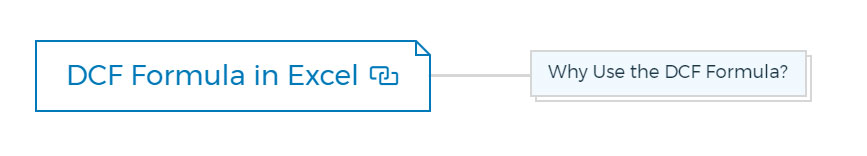### Why Use the DCF Formula?

The use of the DCF formula is to value a company or security. It is the price an investor is willing to pay for an investment given a required rate of return (the discount rate).

The DCF formula is used for various purposes, such as -

• To value a company's idea or investment
• To value a firm's stock
• To value a rental property
• To value everything that generates cash flows

## Major components of discounted cash flow analysis

There are three primary Discounted Cash Flow Analysis components -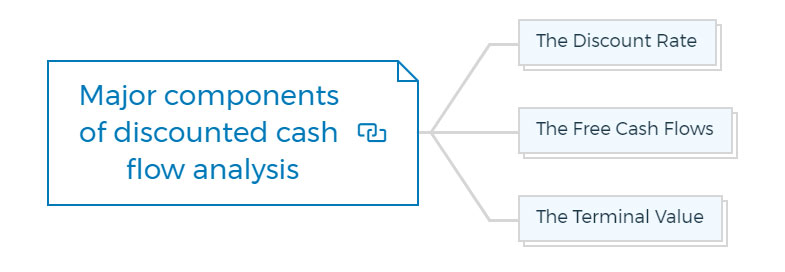### The Discount Rate

The Discount Rate is the return that an investor requires on their investment. This rate will be higher if the investment is riskier. For example, a government bond will have a lower Discount Rate than a stock.

The Discount Rate is also known as the "hurdle rate" or the "required rate of return."

### The Free Cash Flows

The Free Cash Flows are the cash flows available to all investors, including debt and equity holders. These are also known as "unlevered free cash flow".

### The Terminal Value aka Sale Value

The Terminal Value is the value of a company at the end of the Discounted Cash Flow Analysis period. You can understand it as the "sell price" or "exit multiple."

There are two methods for calculating the Terminal Value -

• The Multiplier Method
• The Perpetuity Growth Method.

The Discounted Cash Flow Analysis is a powerful tool that you can use to value a company or investment. However, it is important to remember that this is just one valuation method and that there are other factors to consider when making an investment decision.

## Discounted cash flow analysis for an investment property

The first step in the DCF analysis process is the estimation of the individual cash flows. There are two categories of these cash flows:

Operating expenses, which include things like property taxes, insurance, and repairs and maintenance; and

Net operating income (NOI) is the property's rental income minus its operating expenses.

Let's understand the estimation of cash flows in detail.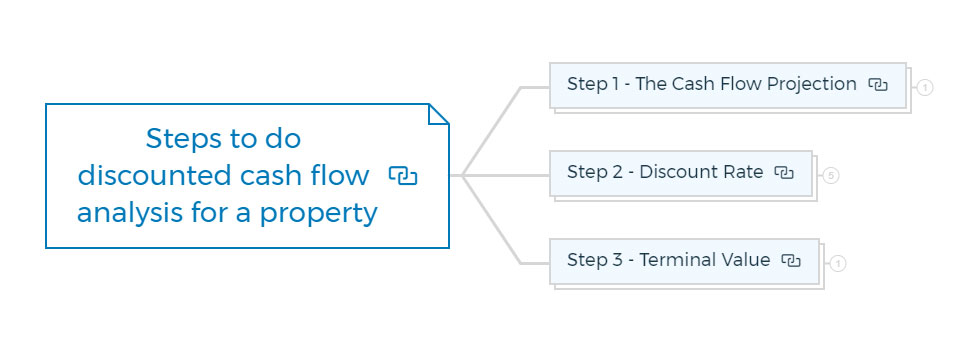### Step 1 - The Cash Flow Projection

Discounted cash flow analysis relies heavily on the definition of the cash flow stream. Most people use free cash flow or net cash flow. These terms are usually defined as follows:

• projected adjusted income after taxes;
• plus reported depreciation and amortisation;
• less necessary capital expenditures;
• less necessary working capital increases;
• and less debt principal repayments, sometimes adjusted for new debt issuance.

Free cash flow is the sum of cash sources less the capital expenditure required to stay in the company and grow at the desired rate. You must cover these costs since a company can't stay in operation if its capital equipment becomes obsolete and can't grow without more working capital investment.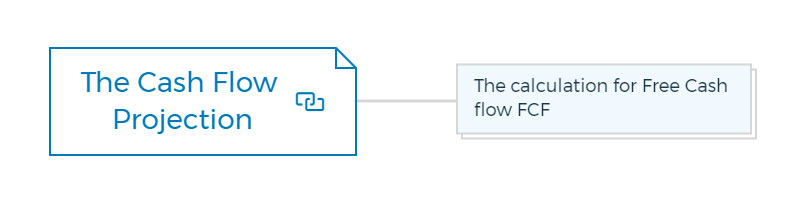#### The calculation for Free Cash Flow FCF

You can calculate free cash flows in three ways

1. Free cash flows formula using operating cash flow2. Free cash flow formula using sales revenue3. Free cash flow formula using net operating profitsThe purpose is to forecast recurrent operating earnings and all cashflow elements related to those earnings, such as essential capital expenditure. The first area of subjectivity in the DCF valuation approach is these estimates.

• Using the asset's financial history as a projection base is one technique to estimate annual cash flows.
• The second area of adjustment is contracts with linked parties. The most prevalent sort of contract in this category is a lease. You can learn more about leases through my article - The Ultimate Guide To Commercial Real Estate Leases.

The land and building are frequently owned by the business owner and leased to the company. Many attempts have been made to bring the lease rate closer to fair market value. However, in some cases, the lease rate is too high; in others, it is too cheap. The arrangement depends on the owner's tax situation.

Appraisers are responsible for adjusting accounting income to account for variations between the actual and economic lease rates. The assumption is that a new buyer might alter the leases (or other forms of contracts) to economical rates, allowing the company's profits to be calculated more correctly.

The third adjustment is to remove income and expenses related to non-operating assets from the income statement, such as an aeroplane that is not used for commercial reasons but is owned and maintained by the company.

To avoid distorting the appraiser's estimate of the company's operating income, eliminate the cost of maintaining and managing this asset from the income statement. Other assets may generate income that must be deducted from the income statement; others may generate income and expenses.

Non Recurring income and expense adjustments are the fourth set of adjustments to make. For example, if a lawsuit costs the firm \$100,000 in legal expenses, and these expenditures are unlikely to recur in the next five to ten years, they should be eliminated.

The capital shortfall is the fifth adjustment an appraiser may be required to make (or surplus). The appraiser must decide if the firm's activities will require more (or less) capital and the impact of adding (or removing) that capital on the income statement.

Finally, adjustments are frequently required to reconcile various accounting procedures. These changes are not only important to make the company's cash flows predictions adhere to the data used to determine the discount rate, but they are also part of the process of calculating the genuine economic income, or cash flow, figures.

Unfortunately, when organisations are publicly owned, it is not always easy to reconcile conflicting accounting techniques. As a result, there is sometimes a clash between these two factors. No modifications should be made in these situations.

The appraiser must ensure that the company's cash flows match those of the publicly traded companies used to calculate the discount rate precisely.

Indeed, an appraiser may be required to prepare two sets of estimated cash flow statements: one that is changed to make the closely held company appear as publicly traded as feasible, and another that strives to represent the economic reality.

### Advanced Property Development Books Bundle

Looking for an edge in the property development game?

This Advanced Property Development Books Bundle is for you. 572+ pages chock-full of insights on residential + commercial property development and investment, this bundle spans 18 ebooks. Whether you're a beginner or a seasoned pro, these books will take your
development game to the next level.7 Real Estate Negotiation Tactics That Win More Deals - 25 pages
7 Easy Steps To Market Your New Development Project - 30 pages
Improve Your Development Game With Development Economics - 40 pages
How To Get Development Approval FAST? - 49 pages
Advanced Real Estate Market Analysis In 13 Easy Steps - 34 pages
Invest In Commercial Property To Achieve Your Investment Goals - 22 pages
10 Finance Options For Your Next Property Development Project - 29 pages
13 Golden Rules Of Investing In Commercial Real Estate - 28 pages
6 Ways To Invest In Commercial Real Estate – The Complete Guide
Commercial Development 101: Become an Expert - 34 pages
Definitive Guide To Commercial Property Investment - 24 pages
A Beginner’s Guide To Apartment Development (Tips & Principles) - 33 pages
How To Build Townhouses And Develop Villas Successfully? - 41 pages
How To Sell A Commercial Property In Record Time? - 25 pages
Raw Land Development - What you need to know - 34 pages
Single Family Homes: Learn Property Development Without Financial Risk - 33 pages
What Exactly Is Equity Finance And How Does It Work? - 42 pages
Real Estate Market – 6 Tips for Timing The Market - 19 pages

### Step 2 - Discount Rate

The discount rate is determined in the second step of the DCF procedure.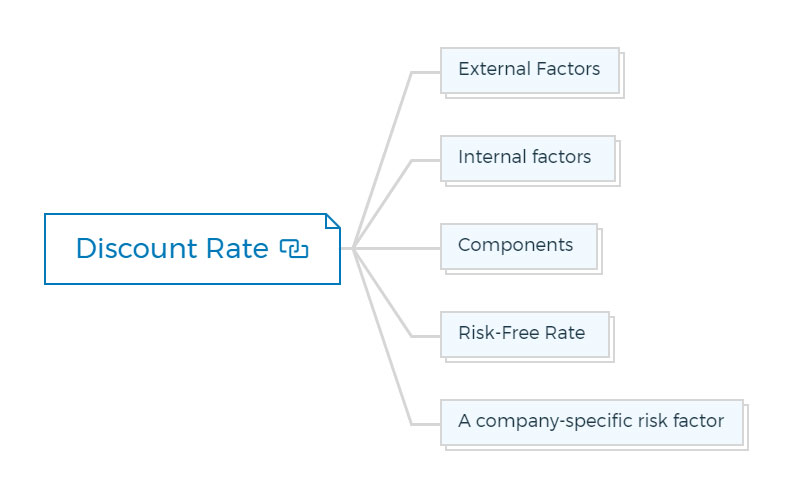Discount Rate Definition

"A discount rate is the rate of return required to entice an investor to participate in the cash flow stream under consideration."

Discount rates have six important aspects. Discount rates -

• Change over time
• The market has an impact on them
• Rely on what's being discounted
• Must be risk-adjusted
• Calculated using various investment yields

The discount rate gets its name because it discounts—that is, lowers the worth of—future quantities in terms of their present value (PV). The following is the formula for this reduction: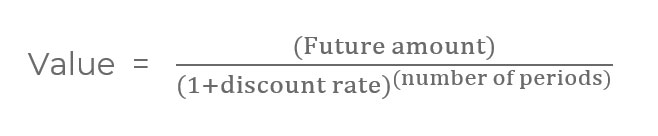These reductions can be huge.

#### External factors

Three main external factors influence discount rates:

• Overall economic conditions
• Alternative investment yields
• Industry conditions and prospects.

The appraiser can get a sense of what can affect the discount rate by studying the external circumstances. Answers to a few simple questions could lead to a wealth of knowledge.

Is the industry, for example, expected to grow at a rate of 5%? What if it's 10%? Is it steady?

Is it becoming smaller?

What impact will the industry's expansion have on cash flows projections?

If the company's management forecasts a 10% annual growth for the next ten years, but the industry is stable or declining, such a forecast may not be accurate.

#### Internal factors

Three internal factors influence the discount rate : (1) financial risk, (2) operating risk, and (3) the risk associated with cash flow stream prediction.

1. There are five primary inputs to financial risk: leverage, coverage, turnover, return, and liquidity. The changes in the discount rate are self-evident; more risk in financial measures must imply higher discount rates.

2. Management, accounting procedures, market stability, customer base, and competitive position are key to operating risk. The appraiser must factor in his judgement of management's ability, whether the accounting processes are prudent or aggressive, and so on when calculating the discount rate.

You should use a greater discount rate if aggressive accounting treatments that raise risk are left in the cash flow predictions. An extremely consistent customer base, on the other hand, may lead to a reduction in the discount rate.

In the same way, if there is a lot of competition, the rate should be greater; if there isn't much rivalry, the rate should be lower.

3. The cash flow stream estimation is fraught with risk. If the 10-year sales and earnings prediction is highly uncertain, as it is in the case of a start-up company, the discount rate must be greater to account for this risk. The increased certainty of the prediction should be reflected in a lower discount rate if the company has a solid operational history and established partnerships.

#### Components

The discount rate consists of three parts:

1. A risk-free rate.
2. A general risk premium that accounts for both equity and industry risk.
3. A company-specific risk factor.

#### Risk-Free Rate

It is the most objectively determined component. Long-term government bonds, intermediate-term Treasury notes, and short-term Treasury bills are the three commonly accepted risk-free rate measurements.

The risk-free instrument that best suits the investment horizon should be used to calculate the discount rate. A 30-year bond could be good for an equity investment with a long time horizon.

Five to seven years is a common financial horizon; intermediate-term notes would be appropriate in this scenario.

Finally, short-term Treasury bills may be the proper estimate of the risk-free rate if an appraiser values something like a patent with only a few years left until expiration.

#### A general risk premium that accounts for both equity and industry risk

Finding the right general risk premium is more difficult for the appraiser. Appraisers estimate this premium using one of four basic ways.

• Direct comparison

It is one of the four regularly utilised strategies. This method entails calculating a discount rate based on real-world transactions.

Unfortunately, obtaining the essential information is frequently difficult.

• The inverse of a price/cash flow ratio with growth

The inverse of a price/cash flow ratio with growth is the second of the four techniques. There are five steps to this procedure.

1. The first stage is to compile a list of publicly traded firms similar enough to the one being appraised to be called comparable, or comparable if you're lucky.
2. The second stage is to calculate price-to-cash-flow ratios for similar publicly traded companies.
3. The third step is to invert the price/cash flow ratio. A capitalisation rate is the same as a cash flow/price ratio. (Note that price = cashflow/cap rate and cap rate= cashflow/price are the same.) Growth is the distinction between a capitalisation rate and a discount rate. The formula is as follows: cap rate + growth = discount rate.
4. The fourth step is to calculate the growth using similar publicly traded companies. It's important to note that the growth rate mentioned here is the expected long-term growth rate (rather than experienced). The growth rate is added to the cash flow/price ratio as the final step in calculating the discount rate.
• Using Stock market return data

Using historical stock market return data is the third way to create a general risk premium. The common source of historical results is the Ibbotson-Sinquefield research.

The difference between stock returns and a risk-free rate is a measure of the additional benefit investors receive for taking on equity risk. The general equity risk premium is the difference between the arithmetic mean return on small-capitalisation stocks and the comparable return on government bonds for appraisal purposes.

• The capital asset pricing model

The capital asset pricing model (CAPM) is the fourth approach for determining the general risk-premium portion of a discount rate. Appraisers may utilise this strategy most frequently. The CAPM is calculated using the following formula:CAPM's required inputs come from a variety of places. Ibbotson and Sinquefield (1989) developed the return on the market (Rm) using historical stock market returns.

Because betas are only available for publicly traded companies, a beta for a private company must be approximated, usually by comparing betas of similar publicly traded companies.

The choice of beta is crucial for calculating a discount rate accurately, yet the appraiser has little control over the process. CAPM theory is also based on expected returns (Rf and Rm) and expected betas calculations.

We can only use historical returns and historical betas in practice. Unfortunately, returns and betas fluctuate with time, making historical measures suspect.

For efficient portfolios, the particular return (alpha) is supposed to be dispersed under the CAPM theoretical framework. However, it is most likely still relevant for particular stocks.

Because the primary inputs are subjective, be cautious of utilising the CAPM to calculate the discount rate. Employing a model like the CAPM to estimate the discount rate makes the process more objective.

Surprisingly, the subjective judgements required in the CAPM process may increase the ultimate discount rate's subjectivity rather than objectivity.

#### A company-specific risk factor

Estimating the company-specific risk premium is the most difficult component of determining a discount rate. Unfortunately, no objective data exists to determine an appropriate company-specific risk premium (or alpha, in CAPM parlance).

Experience is quite beneficial. Each company's condition must be assessed individually, and all risk factors must be identified and factored into the premium. The appraiser must determine a separate beta (or industry beta plus individual alpha) for each subject firm, just as the stock market does for each publicly-traded company.

### Step 3 - Terminal Value

You can calculate the first term in the fair market value estimate equation once the periodic cash flows have been approximated and the discount rate has been determined.

The terminal value is the final information required to complete the discounted cash flow computation. The terminal value would be the enterprise value in year t if it were to be sold in year t.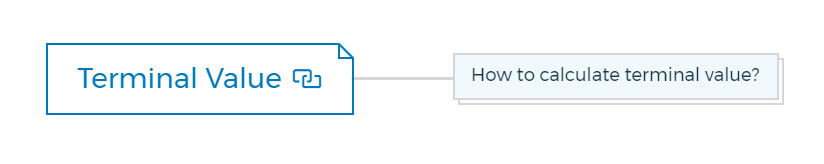#### How to calculate terminal value?

There are a few methods for terminal value calculation for discounted cash flow analysis.

The most popular way is to "capitalise" the predicted cash flow in the following period (period "t + 1"). If the cash flow stream's growth rate is consistent over periods one to infinity in the actual Discounted cash flow analysis formula and the growth is minimal in comparison to the discount rate, the equation simplifies to: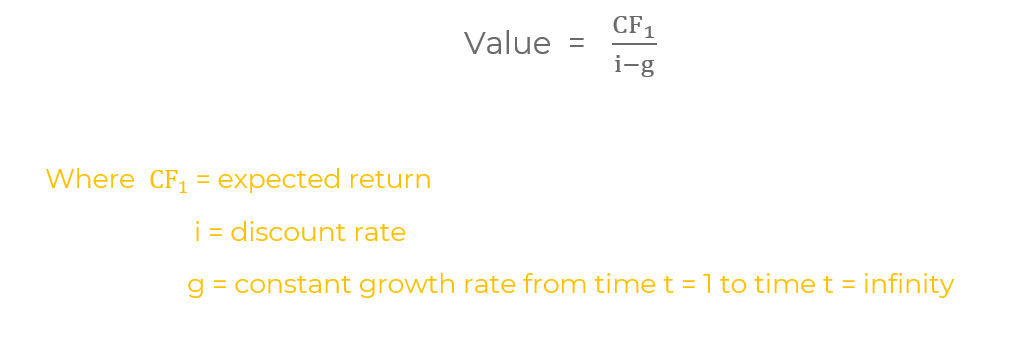In business appraisal, the terminal value formula is -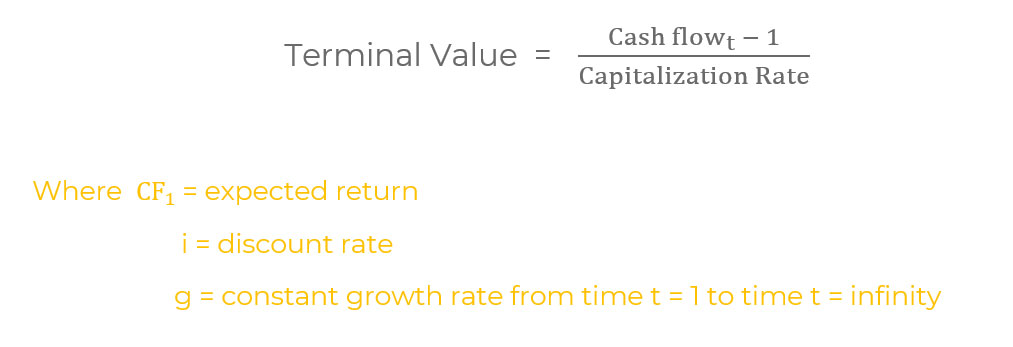The cash flow used in the formula is the cash flow for the following year.

Risk and growth are both factored into the capitalisation rate.

The formula for single-period capitalisation is the same. The cash flow, like the growth, is supposed to endure indefinitely. The terminal value given by the formula is for a date in the future. It must be discounted to the present.

The capitalised terminal value must be discounted to the present value as of the appraisal date, and it must be as of the end of year t (not "t + 1," even if the cash flow must be for the year "t + 1").

The capitalisation rate is a crucial factor to consider. It's pretty uncommon for the residual value phrase to account for 50 to 80 per cent of the projected value in a DCF analysis approach.

Care is also required since, with capitalisation rates typically ranging from 10% to 30%, even a little adjustment in the capitalisation rate can significantly influence value.

Capitalisation rates, like discount rates, are set by the market, fluctuate over time, are dependent on the asset being capitalised, and have a long-term predicted growth rate.

At this point in the DCF process, the standard method for determining a capitalisation rate is to remove an estimate of long-term growth from the discount rate determined earlier. The importance of the growth rate's long-term character cannot be overstated.

If long-term inflation expectations are between 4% and 5%, the growth rate will be between 4% and 5%, implying no actual growth. If there is additional real growth, the analyst must determine how long the company can expand faster than the population growth rate, which is only 1 to 2 per cent, and what the extra rise would average over a 50-year time horizon.

You are missing out if you haven't yet subscribed to our YouTube Channel

## Why use a discounted cash flow method?

Three criteria make the discounted cash flows model the best choice for real estate valuations:

• As a result of opportunity cost, DCF recognises and values the three main aspects of net investment: cash flow, time, and risk.
• The DCF model already dominates real estate investment valuation. Many of you undoubtedly already know it.
• The Discounted cash flow method is relatively efficient and extensively usable in modern spreadsheet applications. Calculations take seconds or less. In uncertain settings, the model can realistically reflect a wide range of complex situations, useful for valuing flexibility.

DCF focuses on cash vs accounting indicators like net income. Of course, accounting measures are vital and Discounted cash flow models frequently include and use them.

Cash is what you can employ in investments, business, and life. Accounting metrics are indirect representations, reflections, or actual or anticipated cash flow forecasts.

The "D" in DCF stands for time and risk. Inflation reduces the value of future cash flows.

1. You may use the money in the meantime (for consumption or investment) and
2. The future cash flows may not materialise in full or at all (no one has a crystal ball).

The discount rate used in the discount cash flows technique to convert future projected cash flows to Present Value accounts for both factors. It uses a discount rate that reflects what an investor could anticipate earning by investing in a similarly risky investment. It is simple, sleek, and intuitive.

The DCF model is not just sound economical, but it is widely used. DCF models use spreadsheets to organise data for value analysis.

Spreadsheets are ubiquitous in finance. Globally, business analysts and decision-makers use spreadsheets like Microsoft Excel. Spreadsheet software is a specific language in business and finance. It can help with communication, understanding, and utilisation.

Finally, DCF models based on computer spreadsheets have considerable analytical range and flexibility, especially in our effort to explicitly include uncertainty and flexibility in valuation.

Spreadsheets allow us to easily update one or more entries and recalculate to see the results instantly. Spreadsheets have two unique features for representing uncertainty and flexibility. These features are simple to set up and utilise.

First, we may utilise spreadsheets to calculate thousands of permutations of the same problem quickly. This feature allows us to cope with a wide range of economic and other variables that may affect an investment's performance and thus its value.

This eliminates the need to limit our analysis to a few conceivable futures. To properly value flexibility, we need to look at probability distributions of possibilities in-depth, such as the effects of economic cycles and market movements. Excel's "data table" and "random number generator" functions provide this feature.

Assemble the spreadsheet to show a decision-maker taking suitable choices under the criteria we provide. We can develop an analogue investment and decision-making model. So we may automate decisions to sell a property in good times or defer construction in bad times.

This skill allows us to express and quantify the benefits of various options and decision flexibility. This method also allows us to simultaneously value several options, which many academic models of option valuation cannot. In Excel calculations, we use "IF statements".

## Mistakes to avoid during DCF analysis

Real estate investors should avoid these three DCF Analysis mistakes:

• Investors often use the wrong Discount Rate when valuing a property. The Discount Rate should be based on the required rate of return for a similar investment.
• Investors often use optimistic assumptions when estimating future cash flows. It is important to be realistic when estimating future cash flows.
• Investors often forget to include all of the costs associated with a real estate investment when estimating future cash flows. Make sure to include all acquisition costs, holding costs, and selling costs when estimating future cash flows.

## Conclusion

In conclusion, discounted cash flow is a powerful financial tool that you can use to make sound investment decisions.

By understanding the components of DCF analysis and using a realistic discount rate, you can make sound projections about a company's future value or investment.

However, it is important to avoid common mistakes when performing a DCF analysis to get the most accurate results.

### Why use discounted cash flow in real estate?

Discounted cash flow (DCF) is the dominant valuation method for real estate investments for a few reasons:
1. It's easily translatable to modern spreadsheet applications.
2. It reflects the time value of money by discounting future cash flows back to the present.
3. It considers the risks associated with an investment, which is especially important when valuing illiquid assets like real estate.

### Are NPV and DCF the same?

No, NPV and DCF are not the same. Discounted Cash Flow (DCF) is a method of valuing a project, company, or asset using the time value of money. Net Present Value (NPV) is the present value of all cash flows associated with an investment. NPV includes positive and negative cash flows, while DCF only includes positive cash flows.